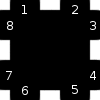*Random Crazy Person Thought of the Day: Ultra-specialized Geomorphs and Naming Conventions*

## Standard Geomorph

A geomorph has 4 sides, and connects to all for sides via two entryways or “ports.” It looks a little bit like an octothorpe/hash with the center filled in (#).## Base2 Geomorph Sets

While Standard Geomorph tiles are cool, theres no way to close the system. To do this, you need to introduce the concept of a side being open (has two connecting ports) or closed (has no connecting ports).

Since there are only two options per side, we can represent each side with a binary number- 0 for closed, 1 for open. By using binary, we can now represent our tile as a four digit binary number. A four digit binary number has 16 possible states (0000, 0001, 0010, 0011, 0100, 0101, 0110, 0111, etc).  If we allow for rotation, we can reduce the total number of unique tiles needed, i.e. 1000, 0100, 0010 and 0001 can all be represented with the same one-sided geomorph by turning it.

With the addition of rotation, we can reduce our 16 down to 6 unique configurations:

```0000= Sealed Geomorph
0001= One-sided Cave Geomorph
0011= Two-sided Corner Geomorph
0101= Two-sided Tunnel Geomorph
0111= Three-sided Edge Geomorph
1111= Four-sided Standard Geomorph```

Suppose we wanted to create store hundreds of tiles with these configurations. How would we store them? The most logical way is to create directories based on their configuration, which could be named after the binary number above. if you needed a eastern wall, you could translate it to 1011, which is simply a three-sided Edge geomorph with a 90 degree clockwise rotation. You could then snag a random one-sized edge tile from 1011/ and simply rotate it.

## Base4 Geomorph Set

While this is neat, you can take it a step further with segmented geomorphs, which track the state of individual left and right ports:

```00= both closed
01= first open
10= second open
11= both open```

The addition of these two new states forces us to use 2 bits to represent state per side, or 8 bits total to represent a tile.This means there are 256 different configurations for tiles. This can be reduced not only by rotation, but by flipping:

```10 00 00 00 = Top left open
01 00 00 00 = Top right open (the above tile, flipped on it's Y axis)```

(Also note, flipping along the X and Y axis has the same effect as rotating 180 degrees.)

```00 10 00 00 = right top open
00 01 00 00 = right bottom open (flipped along X axis)
00 00 00 01 = left top open (flipped along Y axis)
00 00 00 10 = left bottom open (rotated 180 degrees)
00 00 00 10 = left bottom open (flipped along X and Y axis)```

By the time you add in flipping and rotating, we end up with significantly less than 256 tiles. How much? I have no idea, the math is beyond me right now without drawing them all out. What I can say is that we can represent them with Base4 notation:

```0= both closed
1= first open
2= second open
3= both open```

This allows us to represent every tile category with only 4 digits. Looking at what we’ve represented previously:

```0000= Sealed Geomorph
0003= One-sided Cave Geomorph
0033= Two-sided Corner Geomorph
0303= Two-sided Tunnel Geomorph
0333= Three-sided Edge Geomorph
3333= Four-sided Standard Geomorph```

But we could also represent things like:

• a pinwheel configuration: 1111
• a crooked fork in the road:   0302
• a narrow corridor  0102

Among others.

## Base8 Geomorphs

Lets take it another step- lets say that the solid center part between the two ports was changeable, essentially giving us 3 ports; three binary positions giving us a total of 8 combinations per side.

```000 = all closed
001 = right open
010 = center open
011 = center and right open
100 = left open
101 = left and right open  (standard geomorph)
110 = center and left open
111 = all three open```

With 3 bits per side, that gives us a total of 12 bits to represent a geomorph; If I remember my Base2 properly, that’s 4096 possible configurations (again much less with rotation and flips). We could still represent our standard configurations with only 4 digits if we use octal:

```0000= Sealed Geomorph
0005= One-sided Cave Geomorph
0055= Two-sided corner Geomorph
0505= Two-sided Tunnel Geomorph
0555= Three-sided Edge Geomorph
5555= Four-sided Standard Geomorph```

In addition we could create neat things like plusses, crosses, Y’s, trees, etc.

## Base32 Geomorphs

If we wanted to take this one last insane step further, we could introduce the idea of ultra-specialized. where the 2 solid edges of each side were turned into ports. This means there are 5 binary areas (open or closed) per side, which translates to 32 configurations per side, meaning we can use base32 to encode each of the four sides with a simple four-letter code.

To this end, you could represent a “regular” geomorph side with the binary representation, i.e. 01010, which is 10 in decimal and A in base32. This means a regular geomorph tile would be encoded as AAAA.

```0000=sealed Geomorph
A000= One-sided Geomorph
AA00= Two-sided Corner Geomorph
A0A0= Two-sided Tunnel Geomorph
AAA0= Three-sided Edge Geomorph
AAAA= Four-sided Standard Geomorph```

So, the final tally? Five binary on 4 sides is 20 bits of data per tile; That’s over a million different variations. My brain hurts now.

Until I sat down and did the math, I thought 5bit-sided geomorphs were doable. Now I see how wrong I was.